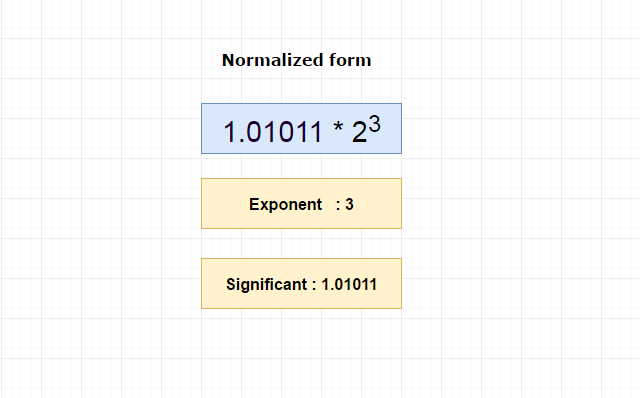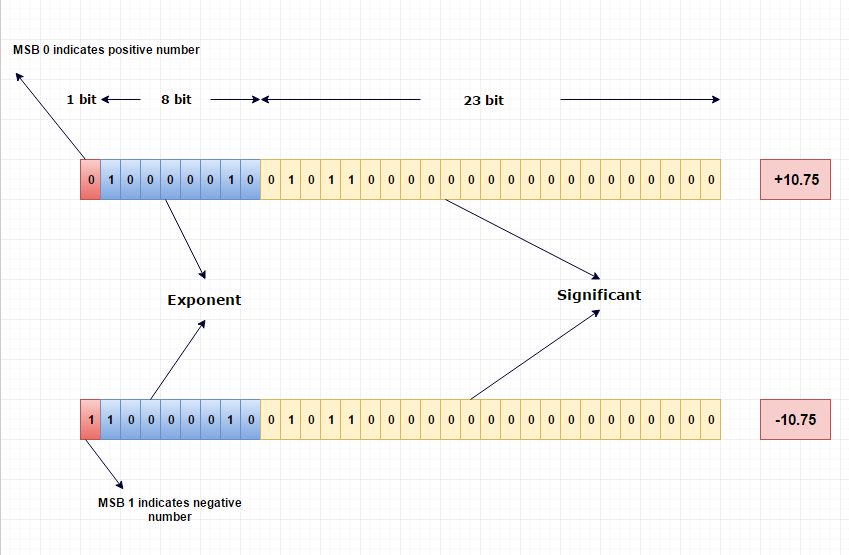How float or double values are stored in memory?

To store a floating-point number, 4-byte(32 bit) memory will be allocated in computer.

1 bit for sign

8 bit for exponent part

23 bit for significant part

Procedure

Let’s discuss the procedure step by step with the example,

1.Floating number will be converted to binary number

This we have discussed already. Convert floating number to binary

Using that procedure, we converted 10.75 to (1010.11) 2

2.Make the converted binary number to normalize form

For floating point numbers, we always normalize it like 1.significant bit * 2 exponent

So, 1010.11 will be normalized as,

1.01011 * 2 3. Since I have shifted 3 bits to left side.

Pictorial ExplanationIn floating number, no concept called 2’s complement to store negative numbers. To overcame that, they came up with bias concept where we add some positive value to negative exponent and make it positive.

In general, whether it negative or positive they add bias value to exponent value to reduce implementation complexity.

Formula to calculate bias value is

biasn = 2n-1 - 1;

Here, we have allocated 8 bits for exponent. So n will be 8

So, 2 7 - 1 = 127

Hence the normalized exponent value will be,

Actual exponent + bias value which is 130 (3 + 127)

Binary form of 130 is (10000010) 2

Representation

Now we have,

Sign bit 0 because 10.75 is positive number

Exponent value is 130 which is (10000010) 2

Significant value is 1.01011, here we can eliminate 1 before the dot (.) because whatever be the number we always going to normalize as 1.something. So, no need to store the 1. Just take bits after the dot (.) which is 01011.

Pictorial ExplanationDouble precision Number - Double

To store double, computer will allocate 8 byte (64 bit) memory.

Where,

1 bit for sign,

11 bit for exponent,

52 bit for significant.

only difference between double and float representation is the bias value.

Here we use 11 bit for exponent.So bias value will be 211 - 1 - 1 i.e 210 - 1 which is 1023.

in the case of double, 1023 will be added to exponent. Remaining procedures are as same as floating representation.

Useful Resources

https://en.wikipedia.org/wiki/IEEE_754